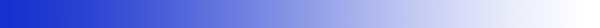MATH401: Green's functions and variational methods Outline of the course and schedule of lectures outline.pdf FINAL EXAM: April 14th 2018, 12:00pm in BUCH B313, duration: 2h30min. Office hours: Thursday 11:00am-12:00pm, with me in my Math office MATH236. By appointment only, so please email me (wachs_at_math.ubc.ca) before if you intend to come. Monday 1:00pm-2:00pm, with Sarafa Adewale Iyaniwura (TA) in LSK300. Same for Sarafa, please email him (iyaniwura_at_math.ubc.ca) before if you intend to come. Lectures notes: Lecture 0: warm up for ODEs Green's functions for ODEs and steady-state PDEs Lecture Green(a): General notions on solving ODEs and PDEs Lecture Green(b): Introduction to generalized functions Lecture Green(c): Green's functions for ODEs Lecture Green(d): Boundary conditions and self-adjoint problems Lecture Green(e): Modified Green's functions and solvability condition Lecture Green(f): Green's functions and eigenfunction expansion Lecture Green(g): Green's functions for PDEs and free-space Green's functions Lecture Green(h): Method of images for simple BCs, solvability condition and modified Green's functions Lecture Green(i): General theory of Green's functions for the Laplacian operator Lecture Green(j): The maximum principle Lecture Green(k): Green's functions by eigenfunction expansion Green's functions for time-dependent PDEs Lecture TD-Green(a): Green's functions for time-dependent PDEs and free-space Green's functions Lecture TD-Green(b): The heat equation: maximum principle, method of images and eigenfunction expansion Lecture TD-Green(c): The wave equation in 1, 2 and 3 dimensions Variational methods Lecture VarMeth(a): Eigenvalue problems and the Rayleigh quotient Lecture VarMeth(b): Bounds on eigenvalues Lecture VarMeth(c): Euler-Lagrange equations Lecture VarMeth(d): Rayleigh-Ritz method Lecture VarMeth(e): Minimization with constraints Assignments: Assignment 1: MATH401_HW1.pdf, due Monday Jan 15th 2018, end of lecture Assignment 2: MATH401_HW2.pdf, due Friday Jan 26th 2018, end of lecture Assignment 3: MATH401_HW3.pdf, due Friday February 9th 2018, end of lecture Assignment 4: MATH401_HW4.pdf, due Wednesday February 13th 2018, end of lecture Assignment 5: MATH401_HW5.pdf, due Wednesday March 14th 2018, end of lecture Assignment 6: MATH401_HW6.pdf, due Wednesday March 21st 2018, end of lecture Assignment 7: MATH401_HW7.pdf, due Wednesday April 4th 2018, end of lecture Assignment 8: MATH401_HW8.pdf, due Friday April 6th, end of lecture, or at the latest April 10th end of the day by email or dropped in the cardboard box in front of my office Solutions to assignments: Assignment 1: MATH401_HW1_solutions.pdf Assignment 2: MATH401_HW2_solutions.pdf Assignment 3: MATH401_HW3_solutions.pdf Assignment 4: MATH401_HW4_solutions.pdf Assignment 5: MATH401_HW5_solutions.pdf Assignment 6: MATH401_HW6_solutions.pdf Assignment 7: MATH401_HW7_solutions.pdf Assignment 8: MATH401_HW8_solutions.pdf Mid-term exam in Spring 2016: Mid-term exam in Spring 2017: